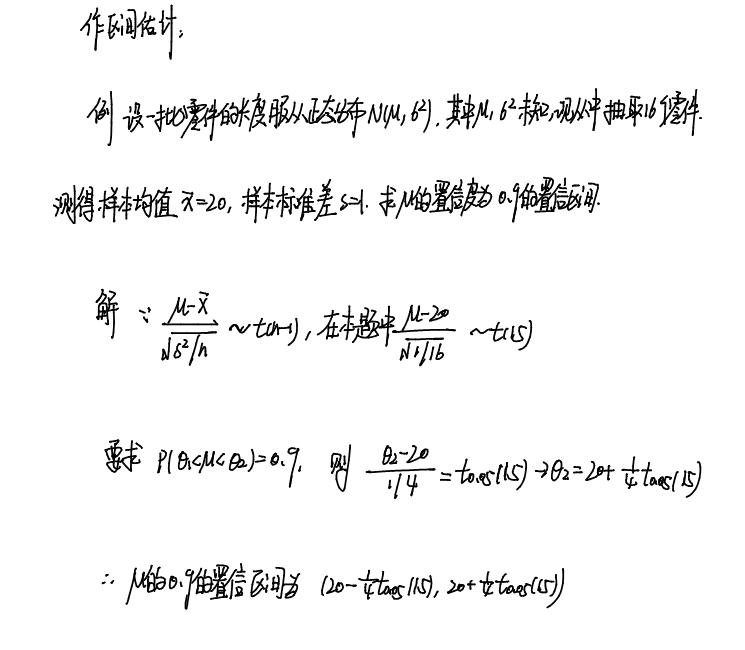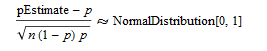区间估计习题

• A+

例子一例子二pEstimate是要估计的值，p可以用频率来代替，n是已知的，这样就可以计算出pEstimate的区间估计了。

``````n = 617519;
m = 317528;
p = m/n;
z = Quantile[NormalDistribution[], {0.005, 0.995}];
ci = p + # Sqrt[(1/n)*p*(1 - p)] & /@ z

>>{0.512561, 0.515838}``````

• 微信公众号
• 关注微信公众号
•• QQ群
• 我们的QQ群号
•